Skip to main content

Thank you for visiting nature.com. You are using a browser version with limited support for CSS. To obtain the best experience, we recommend you use a more up to date browser (or turn off compatibility mode in Internet Explorer). In the meantime, to ensure continued support, we are displaying the site without styles and JavaScript.

# Maximum angle of stability of a wet granular pile

## Abstract

Anyone who has built a sandcastle recognizes that adding liquid to the sand grains increases the overall stability. However, measurements of the stability in wet granular materials often conflict with theory and with each other1,2,3,4,5,6,7. The friction-based Mohr–Coulomb model3,8 distinguishes between granular friction and interparticle friction, but uses the former without providing a physical mechanism. A frictionless model for the geometric stability of dry particles on the surface of a pile2 is in excellent agreement with experiment. However, the same model applied to wet grains overestimates the stability and predicts no dependence on system size. Here we take a frictionless liquid-bridge model and perform a stability analysis within the pile. We reproduce our experimentally observed dependence of the stability angle on system size, particle size and surface tension. Furthermore, we account for past discrepancies in experimental reports by showing that sidewalls can significantly increase the stability of granular material.

## Main

The experimental apparatus consisted of a clear plexiglass drum that was rotated about a horizontal axis. We primarily used a drum with a diameter D of 28.5 cm and a width W that could be varied from 0 to 14.5 cm. A drum with D=12.5 cm and W=11.5 cm was also used, to vary system size. The rotation rate ω was varied from 9.0×10−4 to 5.6×10−1 r.p.m. Soda-lime glass spheres with density ρ of 2.4 g cm−3, radii r=0.25,0.3,0.5 and 1.5 mm and size dispersity within 0.1 mm were used. The drum was 40% filled with grains premixed with a small amount of liquid. We report the amount of liquid added in terms of the volume fraction, Vf, which is defined as the volume of the liquid divided by the volume occupied by the grains alone. The effect of surface tension of the liquid Γ was tested by using silicone oil and water, which have Γ=20±1 and 70±1 dyn cm−1, respectively. Silicone oil with viscosity ν ranging from 5 to 1,000 cS was used to study its impact on the measured inclination angles.

The drum was back lit so that light could pass through a thin layer of grains that tended to accumulate on the sides of the drum, but was unable to pass through the bulk of the pile. Images were acquired with a digital camera of mega-pixel resolution at a rate of three frames per second and these were used to determine the surface slope with automated code. At this rate, the slope of the pile changed by no more than 0.2 between frames. This error in measurement is not significant given that the slope of the pile at the moment of avalanche was distributed over about two degrees in a given run. At least 30 events were recorded to measure the mean values. The error bars in Figs 2 and 4 correspond to the r.m.s. of the mean angles measured over five experimental runs, each consisting of at least 30 avalanche events.

A stick–slip avalanche regime or a continuous avalanche regime is observed depending on the rotation rate of the drum and the liquid viscosity (see Fig. 1). In the stick–slip regime, the slope of the pile increases linearly with time until the heap reaches the maximum angle θm. At this point, the grains are observed to avalanche and the pile’s angle decreases to the angle of repose, θr, when avalanching stops. In the continuous avalanche regime, the grains are observed to flow continuously and the slope of the pile is approximately constant over time. By changing ω and ν, one can change from the stick–slip to the continuous avalanche regime. θm in the stick–slip regime is observed to be independent of ω and ν within the fluctuations in the data and thus is rate independent. On the other hand, θr and the surface angle in the continuous avalanche depend on the viscosity, consistent with other observations6. The overall behaviour as a function of ω is similar to systematic investigations with a rotated drum7, but the magnitudes are significantly lower.Figure 1: Avalanching regimes.

We note that empirically, as a function of Vf, θm increases sharply, and saturates when Vf is of the order of 1×103 θm (see Fig. 2a). The dependence of the angles on Vf is again qualitatively consistent with other studies6,7, but the overall increase and saturation is significantly lower. At very small Vf, θm can be sensitive to Vf because of the inherent roughness of the grains and its impact on the shape of the liquid bridge1. We performed our experiments in the saturation regime, so that such effects were eliminated, but we also chose a small enough Vf so that the liquid did not drain to the bottom because of gravity, which would cause spatial inhomogeneity.Figure 2: Impact of liquid volume fraction and drum width on stability.

We determined experimentally that higher angles can be attributed to significant wall effects when narrow widths are used, as in most other investigations. For example, the angles reported in ref. 10,11 are 15–20 higher than those we report for similar r and Γ, but the apparatus was 3.2 cm wide. The observed dependence on W is plotted in Fig. 2b and is described by an exponential fit. The effect of side walls on grains that are fully immersed in a liquid has been reported in ref. 9. The data in that case reach the asymptotic value for a W of a few grain diameters because liquid bridges are absent. However, the side walls have an effect over many hundred times the diameter of the particle in the partially saturated case because of the added particle–particle correlations induced by the liquid bridges. In order to simplify the analysis, we examine the data only for W>11.5 cm where the side walls are unimportant.

A θm of at least 90 is predicted in ref. 2 if the ratio of the capillary to gravitational force, which is also called the bond number (Bo; ref. 5), is of order one or greater. Now the capillary force caused by the liquid-bridge bond is given by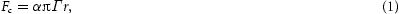where α is a dimensionless constant that depends on the size and shape of the liquid bridge between the particles, and their separation10,11. Although there is some ambiguity in the value of α, it is of order 1, and therefore Bo is approximately 5 for glass beads with r=0.5 mm mixed with silicone oil at Vf=0.008. We do not observe θm to reach 90; the piles typically avalanche when θ exceeds 30 as can be noted from Fig. 1. We believe that the discrepancy arises from the assumption that a wet granular pile fails at the surface, as was also noted in ref. 3.

Following ref. 2, let us consider the stability of a sphere placed on top of three base spheres that are all in contact with each other (see Fig. 3). In the absence of liquid bridges, the sphere becomes unstable when the gravitational force is outside the triangle formed by the base spheres. By averaging over all possible orientation angles φ of the base triangle between 0 and 60, in ref. 2 it was shown that the average inclination θd (the geometric or dry angle of stability) is 23.8. At this angle, approximately half of the triangular bases at the surface can support a sphere and thus the pile will be stable. Measurements with dry glass, plastic or steel spheres in a rotated drum all show θm that are within a few degrees of their prediction, and thus it seems that the complications associated with random packing and friction between particles need not be taken into account to obtain reasonable results.Figure 3: The liquid-bridge model.

Let us again consider a sphere resting on three base spheres (as in Fig. 3b) that is located at a distance x and angle θ from the bottom of the inclined surface. For θ>θd, the sphere will be gravitationally unstable. Now we assume that, on average, it is the component of the liquid-bridge bond ab directed up the inclined plane tilted at θ that is responsible for offsetting the unbalanced gravitation component down the plane. (We neglect the contributions of the bonds ac and ad because they are mostly directed towards the axis around which the top sphere rotates when it becomes unstable.)

Because abcd forms a tetrahedron, the projection of the force Fc up the plane of the base triangle because of the liquid bridge between ab can be easily determined. The average component of the liquid bond force corresponding to the average orientation angle φ of the base triangle is given by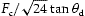, which is approximately 0.46Fc. Therefore, the shear stress that can be supported by the liquid bridges along the θ plane is given by the calculated force per sphere multiplied by the average number of spheres per unit area. Now, the number of particles per unit volume is 3fp/4πr3, where fp is the packing fraction of the grains, which is approximately 0.64 for spherical grains. Therefore, the number of particles per unit area is equal to the number of particles per unit volume raised to the 2/3 power. We then have the shear stress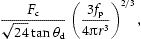where Fc is given by equation (1).

Now let us calculate the shear stress owing to the unbalanced weight of a thin vertical volume element above a plane tilted at θ and located at a distance x from the bottom of the surface, as shown in Fig. 3a. We can write the unbalanced weight as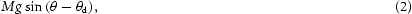where M is the mass of the volume element that has a length dx, width W and a height xsin(θm−θ)/cosθd, and g is the acceleration due to gravity. We take (θ−θd) in equation (2) because the pile is geometrically stable up to θd. Thus we find that the shear stress on the θ plane from the weight of the volume element is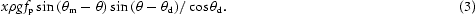To simplify further discussion, we take the small-angle approximation, and obtain the unbalanced stress to be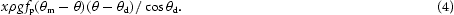Now for each x it can be shown that this shear stress is maximum for θ=(θmd)/2. This is simply because, as one goes above θd, on the one hand there is less material above, but on the other the volume element is tilted further from equilibrium.

It is not clear whether the pile will fail when the shear stress from the weight first exceeds the stress provided by the liquid bridges, or when the average shear stress owing to the weight of the pile exceeds the stress provided by the liquid bridges. In reality, the correct physical situation is most probably a combination of these two. Therefore, we set xL in equation (4) when balancing the stresses, where β is a dimensionless constant between 0.5 and 1, and L is the length of the pile’s surface. If adjacent volume elements cannot offset any stress by a particular volume element then, β=1, and if the stress is evenly distributed along the slip plane then β=0.5. Given the variance seen in the stick–slip events (see Fig. 1a), and the heterogeneity of the pile at the granular level, β may vary from event to event.

Combining these facts and balancing the two stress components, we obtain the condition for equilibrium,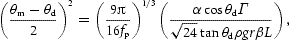and, setting α0=α/β, we obtain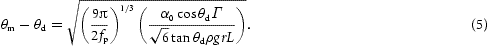Figure 4a shows a comparison of the measured θm as a function of particle size r and the fit to equation (5) after conversion of the units to degrees. The data are well described by using α0=1.2±0.05. To further test the model, we measured θm in two different-sized systems (L=27 and 12.5 cm) with various combinations of Γ and r. Figure 4b shows a plot of the experimentally measured θm above θd compared with equation (5), with α0=1.2. Excellent overall agreement is observed.Figure 4: Comparison of the measured data with the liquid-bridge model.

We note that the small-angle approximation used to simplify equation (3) is reasonable over the angles tested. The analysis can be extended to higher angles without using this approximation, but θm has to be obtained by a numerical solution of the corresponding equation. In developing our analysis, we have ignored the contribution of friction that will also help stabilize the wet pile. However, just as in the dry case, we may expect the friction contribution to be small as a grain rolls after being dislodged from the triangular base. A more detailed knowledge of how the failure develops may clarify this issue.

Our analysis captures the observed stability dependence on grain size, system size and surface tension. It would be of great interest to consider how well this analysis extends to piles composed of non-spherical and multisized grains as in natural sand.

## References

1. 1

Hornbaker, D., Albert, R., Albert, I., Barabási, A. L. & Schiffer, P. What keeps sandcastles standing? Nature 387, 765 (1997).

2. 2

Albert, R., Albert, I., Hornbaker, D., Schiffer, P. & Barabási, A. L. Maximum angle of stability in wet and dry spherical media. Phys. Rev. E 56, 6271–6274 (1997).

3. 3

Halsey, T. C. & Levine, A. J. How sandcastles fall. Phys. Rev. Lett. 80, 3141–3144 (1998).

4. 4

Fraysse, N., Thome, H. & Petit, L. Humidity effects on the stability of a sandpile. Eur. Phys. J. B 11, 615–619 (1999).

5. 5

Nase, S. T., Vargas, W. L., Abatan, A. A. & McCarthy, J. J. Discrete characterization tools for cohesive granular materials. Powder Technol. 116, 214–223 (2001).

6. 6

Samadani, A. & Kudrolli, A. Angle of repose and segregation in cohesive granular matter. Phys. Rev. E 64, 050301 (2001).

7. 7

Tegzes, P., Vicsek, T. & Schiffer, P. Avalanche dynamics in wet granular materials. Phys. Rev. Lett. 89, 094301 (2002).

8. 8

Nedderman, R. M. Statics and Kinematics of Granular Materials (Cambridge Univ. Press, Cambridge, England, 1992).

9. 9

du Pont, S. C., Gondret, P., Perrin, B. & Rabaud, M. Wall effects on granular heap stability. Europhys. Lett. 61, 492–498 (2003).

10. 10

Lian, G., Thornton, C. & Adams, M. J. A theoretical study of the liquid bridge forces between two rigid spherical bodies. J. Colloid Interface Sci. 161, 138–147 (1993).

11. 11

Groger, T., Tuzun, U. & Heyes, D. M. Modeling and measuring of cohesion in wet granular materials. Powder Technol. 133, 203–215 (2003).

Download references

## Acknowledgements

We thank J. Norton and N. Israeloff for their help with the apparatus, and J. Bico for feedback on the manuscript. The work was supported by the National Science Foundation Grant No. DMR-9983659, and the GLUE program of the Department of Energy.

## Author information

Authors

### Corresponding author

Correspondence to Arshad Kudrolli.

## Ethics declarations

### Competing interests

The authors declare no competing financial interests.

## Rights and permissions

Reprints and Permissions

## About this article

### Cite this article

Nowak, S., Samadani, A. & Kudrolli, A. Maximum angle of stability of a wet granular pile. Nature Phys 1, 50–52 (2005). https://doi.org/10.1038/nphys106

Download citation

• Received:

• Revised:

• Accepted:

• Published:

• Issue Date:

## Further reading

• ### Effect of viscosity on the avalanche dynamics and flow transition of wet granular matter

• Jens H. Kasper
• , Vanessa Magnanimo
• , Sjoerd D.M. de Jong
• , Arjan Beek
•  & Ahmed Jarray

Particuology (2021)

• ### Bulk properties variability and interdependency determination for cohesive iron ore

• M. Javad Mohajeri
• , Mats J. van den Bos
• , Cees van Rhee
•  & Dingena L. Schott

Powder Technology (2020)

• ### Air entrainment and granular bubbles generated by a jet of grains entering water

• A.M. Cervantes-Álvarez
• , Y.Y. Escobar-Ortega
• , A. Sauret
•  & F. Pacheco-Vázquez

Journal of Colloid and Interface Science (2020)

• ### Dynamic behavior of humid granular avalanches: Optical measurements to characterize the precursor activity

• Luc Oger
• , Claude el Tannoury
• , Renaud Delannay
• , Yves Le Gonidec
• , Irene Ippolito
• , Yanina Lucrecia Roht
•  & Iñaki Gómez-Arriaran

Physical Review E (2020)

• ### On the Mechanism of Laboratory Earthquake Nucleation Highlighted by Acoustic Emission

• A. A. Ostapchuk
•  & K. G. Morozova

Scientific Reports (2020)

## Search

### Quick linksSign up for the Nature Briefing newsletter — what matters in science, free to your inbox daily.

Get the most important science stories of the day, free in your inbox. Sign up for Nature Briefing